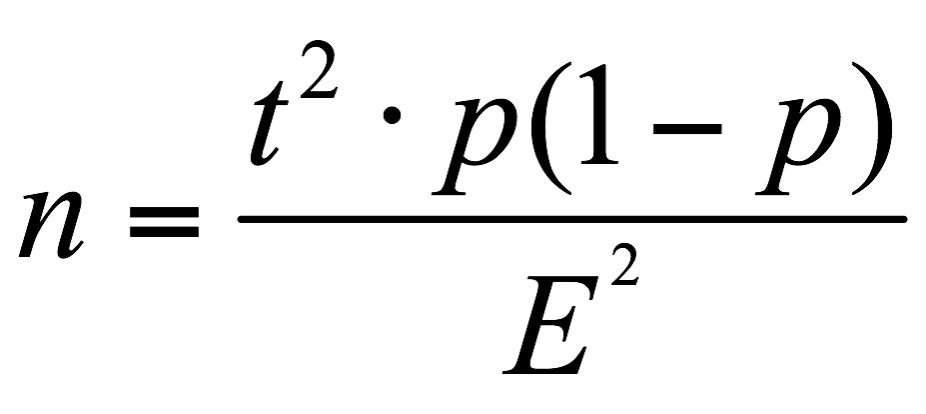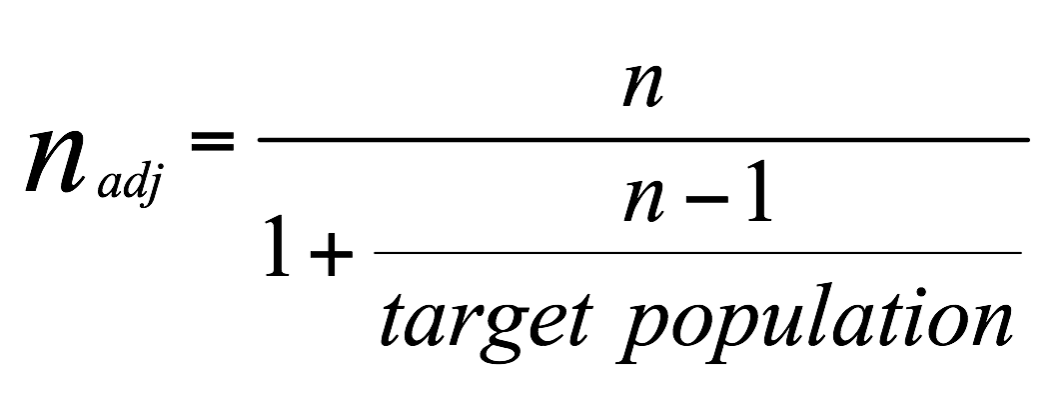Research

# Sampling and Data Collection

## What is sampling?

When a survey has been generated and tested carefully, it is time to send out the survey in order to collect responses. The distribution procedure and data collecting process, is one of the most important parts of the survey processing which has a big influence on further analysis.

Sometimes, even the entire population will be sufficiently small and researcher can consider an obvious way to perform that by sending out the survey to all the possible individuals and then waiting for their responses. This type of research is called a “census study”. Although this method seems to be easy and good, it is not applicable and efficient most of the time. Especially when the target population is huge or simply not entirely reachable.

In order to apply the distribution procedure it is usually needed to use some statistical tools and methods to select a small, but carefully chosen group of potential respondents from the total target population. In statistics these useful methods of choosing a subgroup from a population is referred as “Sampling”.

The goal of sampling is to decrease the cost, save time and reduce the amount of work that it would take to survey the entire target population. Since all further analysis is based on the gathered data, it is necessary to select the samples carefully.

As a general issue in sampling one should consider the following:

• All individuals must have the equal chance to be chosen.
• Sample set should be an appropriate representative of the entire target population.

## Different methods of sampling

There are many different ways and methods of obtaining a sample, however it is possible to classify methods in two different categories according to the statistical nature of them. All the methods can be classified as either “probability” or “non-Probability”.

In probability samples, each member of the entire target population has a known non-zero probability of being selected, while in non-probability sampling, members are selected from the entire population in some nonrandom ways.

### Probability sampling methods

Some of the most famous methods of probability sampling are listed below

#### Random sampling:

This is the most basic and natural probability sampling method. Each and every members of the entire population has the known equal chance of being selected. In case of huge target populations, it is often hard or impossible to identify all the members of the population, so the pool of available subjects becomes biased.

#### Systematic sampling:

In this method, every Nth member is selected from the list of entire population. This method is the easiest probability sampling method which is used frequently in order to select a certain number of individuals from a list (e.g. a computer record)

#### Stratified sampling:

This method has superior to random and systematic sampling due to the less sampling error, and it is used commonly as the main robust probability sampling method. Stratified sampling involves the use of a subset (stratum) of the target population in which the members share one or more common characteristic. As an illustration, stratum might be gender, job title, marital status etc. Then a basic probability method like random sampling may apply in order to select the required number of subjects from each stratum.

### Non-probability sampling:

Although probability sampling is an appropriate way for selecting target group members, it is not always applicable to apply. In fact many survey research are not based on probability samples, but rather on finding a subset of target population as an appropriate collection of respondents to accomplish the survey. One could consider some of the most common non-probability sampling methods as they listed below.

#### Convenience sampling:

This method is commonly used in exploratory research in order to reveal an approximation of the truth inexpensively. As it is obvious from the title, sample is selected because they are convenient to be selected via selecting whatever persons can be most easily access to accomplish the survey. Convenience sampling is mostly used in preliminary research efforts in order to achieve a gross estimation of results without devoting the required cost and time to gain a random sample.

#### Judgment sampling:

This method is a common non-probability sampling method in which the researcher decides which members of the entire population should be selected based on his/her judgment. Since the researcher’s judgment is the criteria for selecting sample, it is necessary that s/he ensures that the selected sample is an appropriate representative of the entire target population and if it is needed some alternative justification for representativeness may be applied.

#### Quota sampling:

this method is the non-probability version of the stratified sampling i.e. the researcher determine the stratums firstly, then one of the non-probability methods like convenience or judgment sampling will applied in each stratum. The difference between quota sampling and stratified sampling is that the randomness is not included in the first one. This method is widely used in non-probability market research surveys.

#### Snowball sampling:

this method is often used when the suitable sample characteristic is rare. It relies on referrals from a small selected group of the target population to recruit additional members of the entire target population. This method may increase bias by reducing the probability of obtaining the sufficient representativeness.

Generally, in non-probability samples it is not possible to measure the trade-off between the target population and the sample derived from it. Furthermore, the potential bias in sampling is unknowable and not detectable.

## Pitfalls of sampling

The difference between probability sampling and non-probability sampling method is vital and one should understand this discrepancy before implementing the sampling.

Despite probability sampling in which the corresponding probability for all members of the target population is known in advance, non-probability sampling methods require less time and effort apply but generally they do not support statistical inference procedures.

Furthermore, probability sampling methods are highly affected by problem of non-coverage and frame problem. As an illustration, considering online survey system, it is possible that not all the target population access to the internet in order to respond to the survey (non-coverage problem). On the other hand, it is possible that the research suffer from the lack of a complete list of contact information (e.g. email address) of the target population, which leads to frame problem. Since both non-coverage and frame problems have a big influence on data quality, they should be reported when publishing the survey results.

Considering online surveys, researcher commonly publish the survey in the form of sharing the URL link on different media, or simply by publishing it on their own websites in order to overcome to the frame problem. However, it leads to sample selection bias which is almost out of the research control and get rise to the non-probability samples.

The standard statistical inference methods like confidence interval calculation and hypothesis test require a probability sample to be efficient and they may not return a reliable results in case of applying directly on non-probability samples.

However the actual survey practices, e.g. in marketing research and opinion polling in particular, tremendously neglect the principle of the probability sampling. Hence it is recommended to consult with a professional sampling statistician to specify the condition that non-probability sampling may work correctly.

## Sample size determination

From the sampling point of view. It is important to have a large enough population to minimize the sampling error in the estimations. Sampling error can be reduced by increasing the sample size or decreasing the random error in the data collection process.
There are theoretical background and presentation for sample size estimation methods which is out of scope of this article, but one can use the following simple formula in order to determine the required needed sample size.Where t is the confidence level (at 95% it is equal to 1.96),  p is the estimation of the proportion and m is the margin of error.

The margin of error is a statistic describing the amount of random sampling error in a survey’s results. As an example, suppose the margin error is 2% and from all respondents 59% picked an answer. Hence, one can be sure that if the same question had been asked from the entire target population, between 57% and 61% would have pick the same answer.

In order to bringing the size of the entire target population in to account, it is recommended to use the adjusted formula to determine the sample size instead:And at the end, it should be mentioned again that no matter how a survey is designed carefully and efficiently, if the sampling and data gathering process is not done adequately well, the result of the research can be inaccurate, unreliable and ungeneralizable.About the Author

### Pouya Sinaian

Mathematician, statistician data analyst, and lecturer. MSc in "Mathematical Modelling and Simulation" from BTH, Sweden.